Ex 10.3

Chapter 10 Class 11 Straight Lines (Term 1)
Serial order wise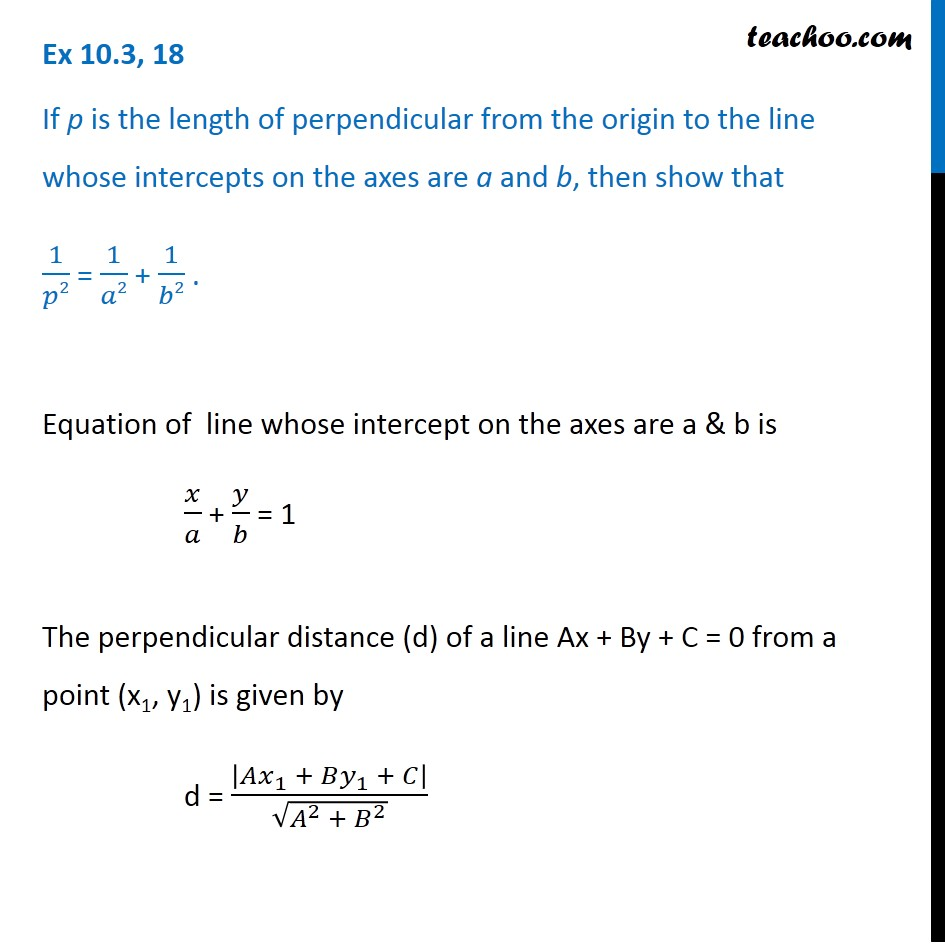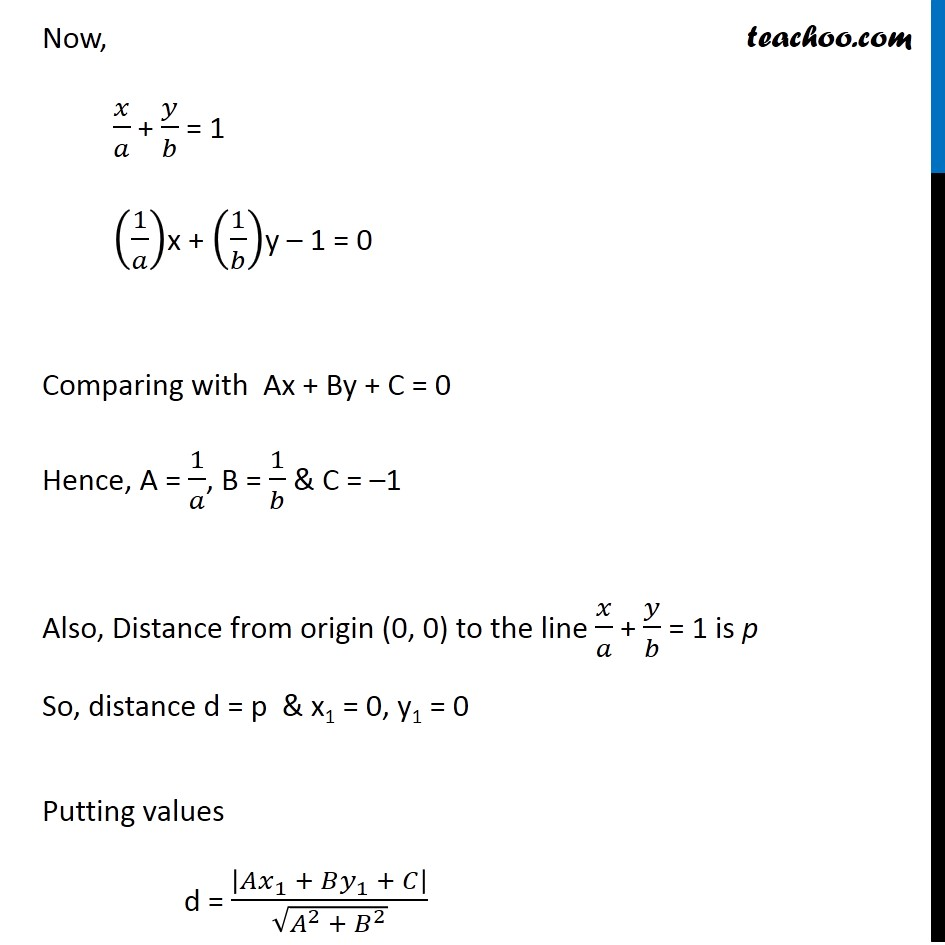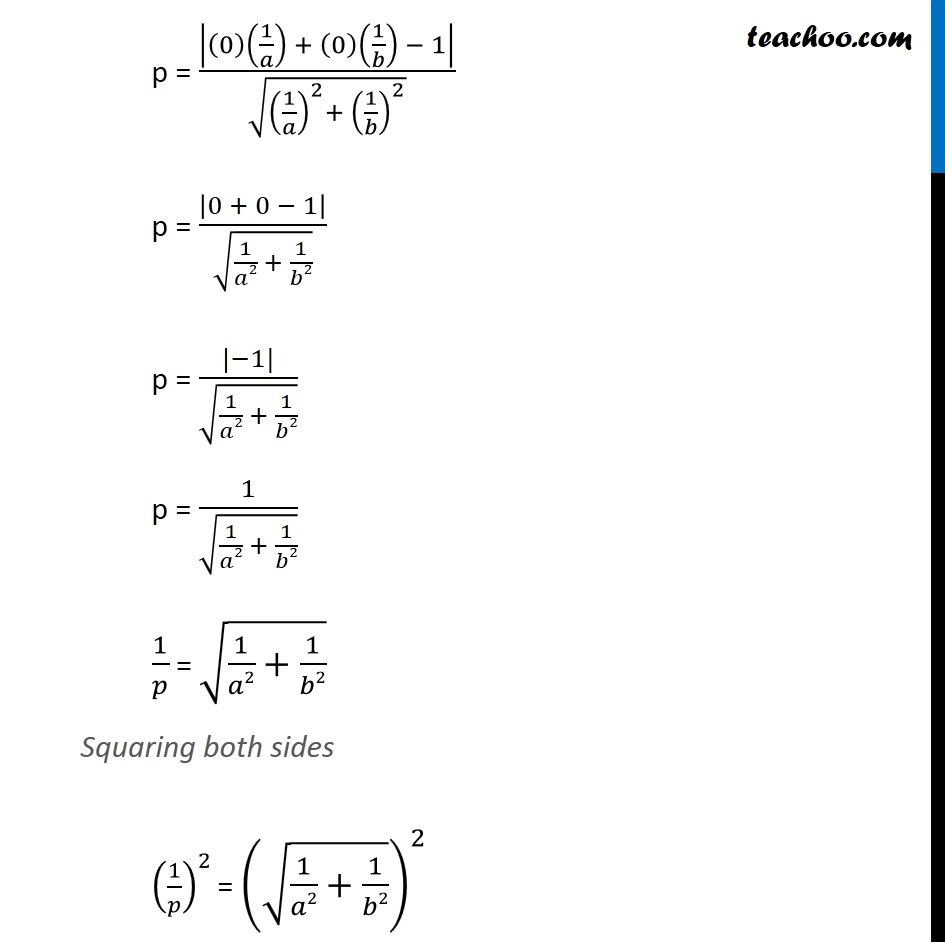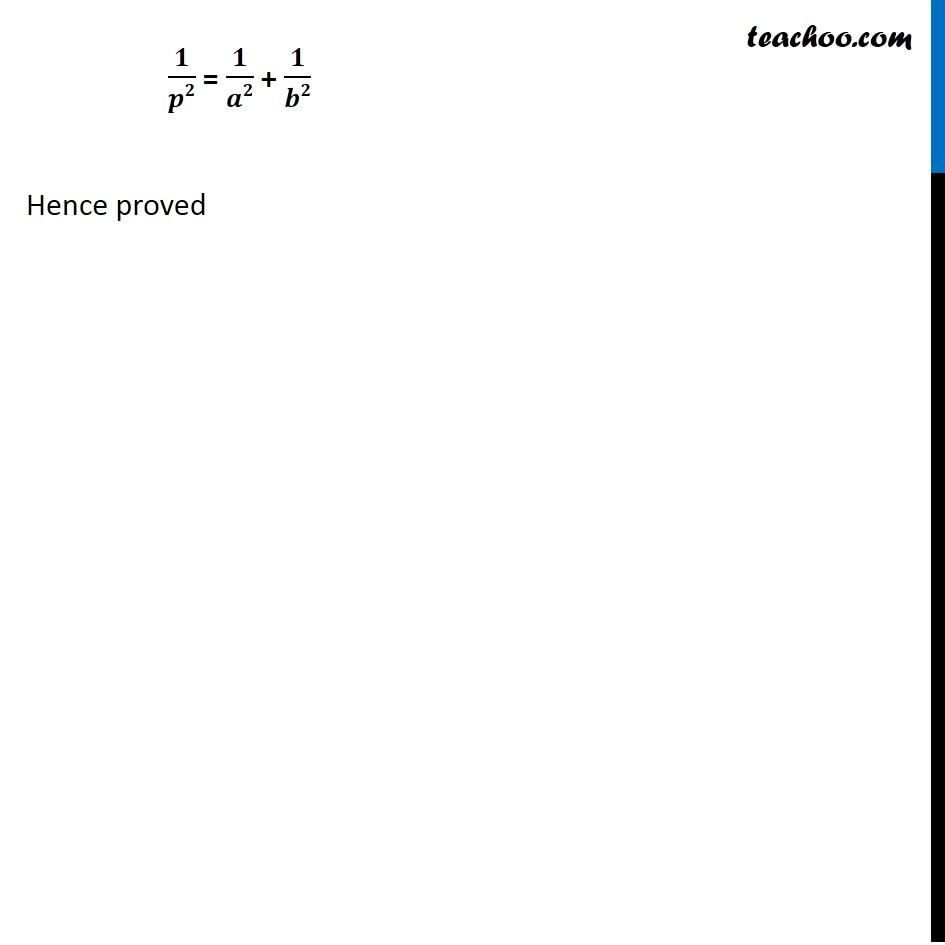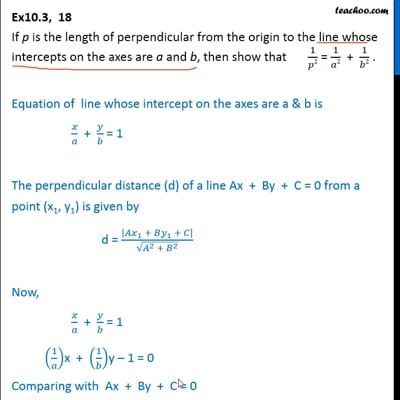This video is only available for Teachoo black users

### Transcript

Ex 10.3, 18 If p is the length of perpendicular from the origin to the line whose intercepts on the axes are a and b, then show that 1/𝑝2 = 1/𝑎2 + 1/𝑏2 . Equation of line whose intercept on the axes are a & b is 𝑥/𝑎 + 𝑦/𝑏 = 1 The perpendicular distance (d) of a line Ax + By + C = 0 from a point (x1, y1) is given by d = |𝐴𝑥_1 + 𝐵𝑦_1 + 𝐶|/√(𝐴^2 + 𝐵^2 ) Now, 𝑥/𝑎 + 𝑦/𝑏 = 1 (1/𝑎)x + (1/𝑏)y – 1 = 0 Comparing with Ax + By + C = 0 Hence, A = 1/𝑎, B = 1/𝑏 & C = –1 Also, Distance from origin (0, 0) to the line 𝑥/𝑎 + 𝑦/𝑏 = 1 is p So, distance d = p & x1 = 0, y1 = 0 Putting values d = |𝐴𝑥_1 + 𝐵𝑦_1 + 𝐶|/√(𝐴^2 + 𝐵^2 ) p = |(0)(1/𝑎) + (0)(1/𝑏) − 1|/√((1/𝑎)^2+ (1/𝑏)^2 ) p = |0 + 0 − 1|/√(1/𝑎2 + 1/𝑏2) p = (|−1|)/√(1/𝑎2 + 1/𝑏2) p = 1/√(1/𝑎2 + 1/𝑏2) 1/𝑝 = √(1/𝑎2+1/𝑏2) Squaring both sides (1/𝑝)^2 = (√(1/𝑎2+1/𝑏2))^2 𝟏/𝒑𝟐 = 𝟏/𝒂𝟐 + 𝟏/𝒃𝟐 Hence proved